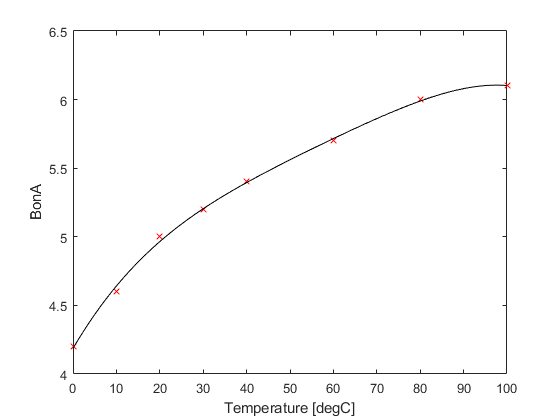waterNonlinearity

Calculate B/A of water with temperature.

Syntax

BonA = waterNonlinearity(T)

Description

waterNonlinearity calculates the parameter of nonlinearity B/A at a given temperature using a fourth-order polynomial fitted to the data given by Beyer (1960, Parameter of nonlinearity in fluids, J. Acoust. Soc. Am., 32(6), 719-721). A plot of the original data and the polynomial fit is given below.Inputs

 T water temperature in the range 0 to 100 [degC]

Outputs

 BonA parameter of nonlinearity